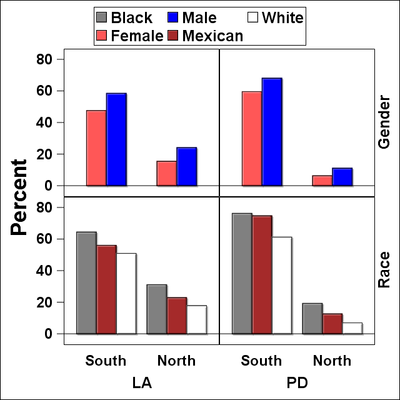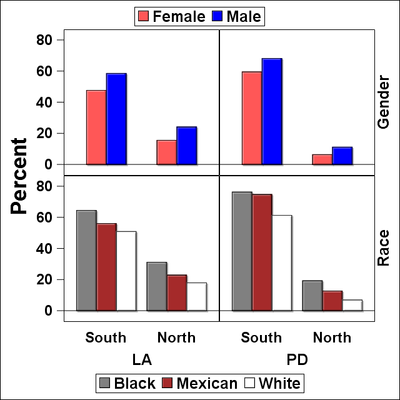## Create separate keylegends in sgpanel

I would like to have 2 keylegends in a 2-panel graph in Proc SGPANEL (see code below). As you may note in the figure below, the keylegends is not sorted by the demographics1 or demographics2. Sorting the data by these 2 variables did not make a difference. Is there any way to make 2 separate legends by the variable demographics1: one for the gender, and the other for race?

Thank you.

proc sgpanel;

styleattrs datacolors=(blue lightred grey brown white)

datalinepatterns=(solid);

vbarparm category=threshold response=prevalence /

group=Demographics2 groupdisplay=cluster

colaxis discreteorder=data

display=(noticks nolabel)

valueattrs=(size=16pt weight=bold);

rowaxis label='Percent'

labelattrs=(size=24pt weight=bold)

valueattrs=(size=16pt weight=bold)

values=(0 to 80 by 20);

keylegend /position=top autoitemsize down=3

title="" sortorder=ascending

titleattrs=(Family=Arial Size=12 Weight=Bold)

valueattrs=(Family=Arial Size=18 Weight=Bold);

run;1 ACCEPTED SOLUTION

Accepted SolutionsDanH_sas
SAS Super FREQ

## Re: Create separate keylegends in sgpanel

Try this EXCLUDE trick in the KEYLEGEND statement and see if it works for you:

``````proc sgpanel;
styleattrs datacolors=(blue lightred grey brown white)
datalinepatterns=(solid);
vbarparm category=threshold response=prevalence /
group=Demographics2 groupdisplay=cluster name="bar"
colaxis discreteorder=data
display=(noticks nolabel)
valueattrs=(size=16pt weight=bold);
rowaxis label='Percent'
labelattrs=(size=24pt weight=bold)
valueattrs=(size=16pt weight=bold)
values=(0 to 80 by 20);
keylegend "bar" / position=topleft autoitemsize across=1
title="" sortorder=ascending
exclude=("Female" "Male")
titleattrs=(Family=Arial Size=12 Weight=Bold)
valueattrs=(Family=Arial Size=18 Weight=Bold);
keylegend "bar" / position=topright autoitemsize across=1
title="" sortorder=ascending
exclude=("Black" "Mexican" "White")
titleattrs=(Family=Arial Size=12 Weight=Bold)
valueattrs=(Family=Arial Size=18 Weight=Bold);
run;``````
3 REPLIES 3DanH_sas
SAS Super FREQ

## Re: Create separate keylegends in sgpanel

Try this EXCLUDE trick in the KEYLEGEND statement and see if it works for you:

``````proc sgpanel;
styleattrs datacolors=(blue lightred grey brown white)
datalinepatterns=(solid);
vbarparm category=threshold response=prevalence /
group=Demographics2 groupdisplay=cluster name="bar"
colaxis discreteorder=data
display=(noticks nolabel)
valueattrs=(size=16pt weight=bold);
rowaxis label='Percent'
labelattrs=(size=24pt weight=bold)
valueattrs=(size=16pt weight=bold)
values=(0 to 80 by 20);
keylegend "bar" / position=topleft autoitemsize across=1
title="" sortorder=ascending
exclude=("Female" "Male")
titleattrs=(Family=Arial Size=12 Weight=Bold)
valueattrs=(Family=Arial Size=18 Weight=Bold);
keylegend "bar" / position=topright autoitemsize across=1
title="" sortorder=ascending
exclude=("Black" "Mexican" "White")
titleattrs=(Family=Arial Size=12 Weight=Bold)
valueattrs=(Family=Arial Size=18 Weight=Bold);
run;``````

## Re: Create separate keylegends in sgpanel

Hi Dan,

It actually worked. I did however get an error message, that "topright" and "topleft" were not acceptable, so I used "top" and "bottom" instead. Here is how the figure looks now.

Thank you very much for your help.DanH_sas
SAS Super FREQ

## Re: Create separate keylegends in sgpanel

Ah, yes...sorry about that. Those values are supported in SGPLOT, but not SGPANEL. The way you have them arranged looks better anyway :-).

Discussion stats
• 3 replies
• 268 views
• 2 likes
• 2 in conversation